# seapy.components.plate.SubsystemBend¶

class seapy.components.plate.SubsystemBend(name, system, **properties)[source]

Subsystem for bending waves in a 2D isotropic component.

__init__(name, system, **properties)

Constructor.

Parameters: name (string) – Identifier component (SeaPy.components.Component) – Component

Methods

 __init__(name, system, **properties) Constructor. addExcitation(name, model, **properties) Add excitation to subsystem. disable([couplings]) Disable this subsystem. enable([couplings]) Enable this subsystem. info([attributes]) Return dataframe. plot(quantity[, yscale]) Plot quantity.

Attributes

 SORT str(object=’‘) -> str average_frequency_spacing Average frequency spacing for bending waves in a 2D isotropic plate. classname Name of class of the object. component conductance Conductance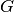. conductance_point_average Average point conductance of a structural component. damping_term The damping term is the ratio of the modal half-power bandwidth to the average modal frequency spacing. dlf Damping loss factor of subsystem. enabled Switch indicating whether the object is enabled. energy Total energy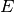in subsystem. flexural_rigidity Flexural rigidity of a plate. frequency Frequency. impedance Impedance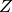impedance_point_force Point impedance. included Indicates whether the object is included in the analysis. linked_couplings_from linked_couplings_to linked_excitations mobility Mobility Y modal_density Modal density. modal_energy Class capable of containing spectral values. modal_overlap_factor Modal overlap factor. name power_input Total input power due to excitations. resistance Resistance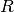, the real part of the impedance. resistance_point_average Average point resistance. soundspeed_group Group velocity for bending wave. soundspeed_phase Phase velocity for bending wave. tlf Total loss factor. velocity Vibrational velocity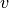. velocity_level Velocity level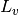. wavenumber Wavenumber of flexural waves in a plate.
average_frequency_spacing[source]

Average frequency spacing for bending waves in a 2D isotropic plate.

Return type: numpy.ndarray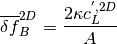with:

• radius of gyration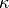• soundspeed of longitudinal waves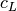• plate area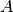See Lyon, eq 8.2.5

flexural_rigidity[source]

Flexural rigidity of a plate.

Return type: numpy.ndarray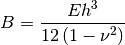with:

• Young’s modulus• plate thickness/height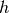• Poisson’s ratio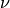See Craik, equation 3.2, page 48.

impedance_point_force[source]

Point impedance.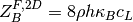with:

• density of the plate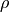• radius of gyration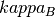• soundspeed of longitudinal wavesSee Lyon, page 201, table 10.1

soundspeed_group[source]

Group velocity for bending wave.

Return type: numpy.ndarray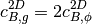See Lyon, above eq. 8.2.5

soundspeed_phase[source]

Phase velocity for bending wave.

Return type: numpy.ndarray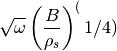with:

• Angular frequency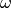• Bending stiffness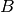• surface density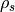See Craik, equation 5.19, page 128.

Note that this is the same as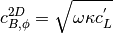See Lyon, above eq. 8.2.5

wavenumber[source]

Wavenumber of flexural waves in a plate.

Return type: numpy.ndarray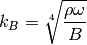with:

• density of the plate• angular frequency• flexural rigidity

System Message: WARNING/2 (\B)

latex exited with error [stdout] This is pdfTeX, Version 3.14159265-2.6-1.40.15 (TeX Live 2014/Debian) (preloaded format=latex) restricted \write18 enabled. entering extended mode (./math.tex LaTeX2e <2014/05/01> Babel <3.9k> and hyphenation patterns for 79 languages loaded. (/usr/share/texlive/texmf-dist/tex/latex/base/article.cls Document Class: article 2007/10/19 v1.4h Standard LaTeX document class (/usr/share/texlive/texmf-dist/tex/latex/base/size12.clo)) (/usr/share/texlive/texmf-dist/tex/latex/base/inputenc.sty (/usr/share/texlive/texmf-dist/tex/latex/ucs/utf8x.def)) (/usr/share/texlive/texmf-dist/tex/latex/ucs/ucs.sty (/usr/share/texlive/texmf-dist/tex/latex/ucs/data/uni-global.def)) (/usr/share/texlive/texmf-dist/tex/latex/amsmath/amsmath.sty For additional information on amsmath, use the `?’ option. (/usr/share/texlive/texmf-dist/tex/latex/amsmath/amstext.sty (/usr/share/texlive/texmf-dist/tex/latex/amsmath/amsgen.sty)) (/usr/share/texlive/texmf-dist/tex/latex/amsmath/amsbsy.sty) (/usr/share/texlive/texmf-dist/tex/latex/amsmath/amsopn.sty)) (/usr/share/texlive/texmf-dist/tex/latex/amscls/amsthm.sty) (/usr/share/texlive/texmf-dist/tex/latex/amsfonts/amssymb.sty (/usr/share/texlive/texmf-dist/tex/latex/amsfonts/amsfonts.sty)) (/usr/share/texlive/texmf-dist/tex/latex/tools/bm.sty) (./math.aux) (/usr/share/texlive/texmf-dist/tex/latex/ucs/ucsencs.def) (/usr/share/texlive/texmf-dist/tex/latex/amsfonts/umsa.fd) (/usr/share/texlive/texmf-dist/tex/latex/amsfonts/umsb.fd) ! Undefined control sequence. <recently read> \B l.12 $\B$  (./math.aux) ) (see the transcript file for additional information) Output written on math.dvi (1 page, 152 bytes). Transcript written on math.log.

See Langley and Heron, 1990, eq 18.

#### Previous topic

seapy.components.plate.SubsystemLong

#### Next topic

seapy.components.plate.SubsystemShear